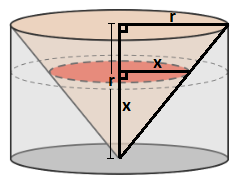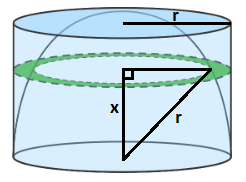# Brain Teaser

Consider the two shapes inside the two identical cylinders whose radius is equal to its height.

The volume on the left is a regular cone inscribed in the cylinder. The volume on the right is formed by subtracting an inscribed hemisphere from the cylinder.

When cut horizontally at the same height from the bottom, which cross-section has a larger area, the red or the green one?• The orange cross-section is larger.
• The green cross-section is larger.
• The cross-sections have the same area.
• The relationship changes depending on the height of the slice.

Let x be the height of the cross-section for both the inscribed cone and hemisphere, and let r be the radius of the cylinder.

The red cross-section is the circular base of a cone that is similar to that of the inscribed cone, and since the inscribed cone has the same radius and height, then by similar triangles the cone with the red cross-section also has the same radius and height, which is x. Therefore, the area of the red cross-section, as a circle with radius x, is Ar =– at any height x .The green cross-section is a ring formed by the difference of the cross-section of the cylinder and the cross-section of the hemisphere. Using the height x of the cross-section as a leg and the radius r of the hemisphere as a hypotenuse, the radius of the circular cross-section of the hemisphere isby Pythagorean’s Theorem.

Therefore, the area of the green cross-section, as a ring with an outer radius of r and an inner radius radius, is  Ag ==at any height x.Since  Ar = Ag =at any height, the cross-sections have the same area.

Note: It should be pointed out for some of the other solutions that the converse of Calvieri’s Principle (that if the solids have the same volume, then they must have the same cross-sectional area) is not necessarily true. A counter-example would be to turn the inscribed cone in the given problem upside down – then the cross-sections at the very bottom of the cylinder would be a full circle for the cone but O  for the hemisphere.

• Problem by BRILLIANT
• Solution by David Vreken
• Powered by